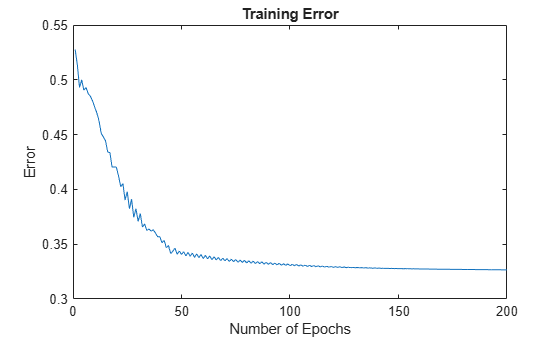# Model Suburban Commuting Using Subtractive Clustering

This example shows how to model the relationship between the number of automobile trips generated from an area and the demographics of the area using the `genfis` function. Demographic and trip data are from 100 traffic analysis zones in New Castle County, Delaware. Five demographic factors are considered: population, number of dwelling units, vehicle ownership, median household income, and total employment. Hence, the model has five input variables and one output variable.

```mytripdata subplot(2,1,1) plot(datin) ylabel('input') subplot(2,1,2) plot(datout) ylabel('output')```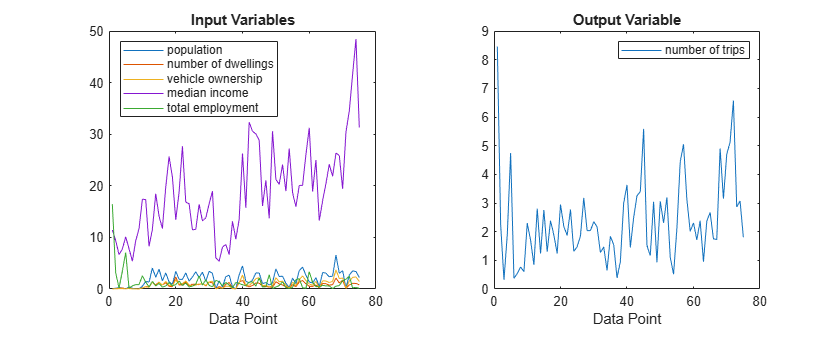The `mytripdata` command creates several variables in the workspace. Of the original 100 data points, use 75 data points as training data (`datin` and `datout`) and 25 data points as checking data (as well as for test data to validate the model). The checking data input/output pair variables are `chkdatin` and `chkdatout`.

Generate a model from the data using subtractive clustering using the `genfis` command.

First, create a `genfisOptions` option set for subtractive clustering, specifying `ClusterInfluenceRange` range property. The `ClusterInfluenceRange` property indicates the range of influence of a cluster when you consider the data space as a unit hypercube. Specifying a small cluster radius usually yields many small clusters in the data, and results in many rules. Specifying a large cluster radius usually yields a few large clusters in the data, and results in fewer rules.

`opt = genfisOptions('SubtractiveClustering','ClusterInfluenceRange',0.5);`

Generate the FIS model using the training data and the specified options.

`fismat = genfis(datin,datout,opt);`

The `genfis` command uses a one-pass method that does not perform any iterative optimization. The model type for the generated FIS object is a first order Sugeno model with three rules.

Verify the model. Here, `trnRMSE` is the root mean squared error of the system generated by the training data.

```fuzout = evalfis(fismat,datin); trnRMSE = norm(fuzout-datout)/sqrt(length(fuzout))```
```trnRMSE = 0.5276 ```

Next, apply the test data to the FIS to validate the model. In this example, the validation data is used for both checking and testing the FIS parameters. Here, `chkRMSE` is the root mean squared error of the system generated by the validation data.

```chkfuzout = evalfis(fismat,chkdatin); chkRMSE = norm(chkfuzout-chkdatout)/sqrt(length(chkfuzout))```
```chkRMSE = 0.6179 ```

Plot the output of the model, `chkfuzout`, against the validation data, `chkdatout`.

```figure plot(chkdatout) hold on plot(chkfuzout,'o') hold off```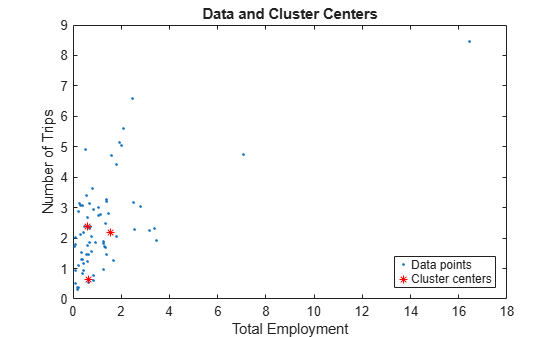The model output and validation data are shown as circles and solid blue line, respectively. The plot shows that the model does not perform well on the validation data.

At this point, you can use the optimization capability of `anfis` to improve the model. First, try using a relatively short training period (20 epochs) without using validation data, and then test the resulting FIS model against the testing data.

```anfisOpt = anfisOptions('InitialFIS',fismat,'EpochNumber',20,... 'InitialStepSize',0.1); fismat2 = anfis([datin datout],anfisOpt);```
```ANFIS info: Number of nodes: 44 Number of linear parameters: 18 Number of nonlinear parameters: 30 Total number of parameters: 48 Number of training data pairs: 75 Number of checking data pairs: 0 Number of fuzzy rules: 3 Start training ANFIS ... 1 0.527607 2 0.513727 3 0.492996 4 0.499985 5 0.490585 6 0.492924 7 0.48733 Step size decreases to 0.090000 after epoch 7. 8 0.485036 9 0.480813 10 0.475097 Step size increases to 0.099000 after epoch 10. 11 0.469759 12 0.462516 13 0.451177 14 0.447856 Step size increases to 0.108900 after epoch 14. 15 0.444356 16 0.433904 17 0.433739 18 0.420408 Step size increases to 0.119790 after epoch 18. 19 0.420512 20 0.420275 Designated epoch number reached --> ANFIS training completed at epoch 20. Minimal training RMSE = 0.420275 ```

After the training is complete, validate the model.

```fuzout2 = evalfis(fismat2,datin); trnRMSE2 = norm(fuzout2-datout)/sqrt(length(fuzout2))```
```trnRMSE2 = 0.4203 ```
```chkfuzout2 = evalfis(fismat2,chkdatin); chkRMSE2 = norm(chkfuzout2-chkdatout)/sqrt(length(chkfuzout2))```
```chkRMSE2 = 0.5894 ```

The model has improved a lot with respect to the training data, but only a little with respect to the validation data. Plot the improved model output obtained using `anfis` against the testing data.

```figure plot(chkdatout) hold on plot(chkfuzout2,'o') hold off```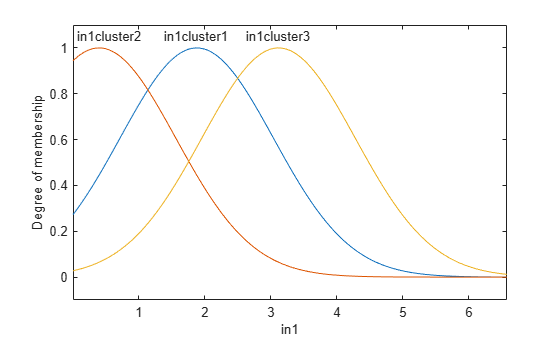The model output and validation data are shown as circles and solid blue line, respectively. This plot shows that subtractive clustering with `genfis` can be used as a standalone, fast method for generating a fuzzy model from data, or as a preprocessor to determine the initial rules for `anfis` training. An important advantage of using a clustering method to find rules is that the resultant rules are more tailored to the input data than they are in a FIS generated without clustering. This result reduces the problem of an excessive propagation of rules when the input data has a high dimension.

Overfitting can be detected when the checking error starts to increase while the training error continues to decrease.

To check the model for overfitting, use `anfis` with validation data to train the model for 200 epochs.

First configure the ANFIS training options by modifying the existing `anfisOptions` option set. Specify the epoch number and validation data. Since the number of training epochs is larger, suppress the display of training information to the Command Window.

```anfisOpt.EpochNumber = 200; anfisOpt.ValidationData = [chkdatin chkdatout]; anfisOpt.DisplayANFISInformation = 0; anfisOpt.DisplayErrorValues = 0; anfisOpt.DisplayStepSize = 0; anfisOpt.DisplayFinalResults = 0;```

Train the FIS.

`[fismat3,trnErr,stepSize,fismat4,chkErr] = anfis([datin datout],anfisOpt);`

Here,

• `fismat3` is the FIS object when the training error reaches a minimum.

• `fismat4` is the snapshot FIS object when the validation data error reaches a minimum.

• `stepSize` is a history of the training step sizes.

• `trnErr` is the RMSE using the training data

• `chkErr` is the RMSE using the validation data for each training epoch.

After the training completes, validate the model.

```fuzout4 = evalfis(fismat4,datin); trnRMSE4 = norm(fuzout4-datout)/sqrt(length(fuzout4))```
```trnRMSE4 = 0.3393 ```
```chkfuzout4 = evalfis(fismat4,chkdatin); chkRMSE4 = norm(chkfuzout4-chkdatout)/sqrt(length(chkfuzout4))```
```chkRMSE4 = 0.5834 ```

The error with the training data is the lowest thus far, and the error with the validation data is also slightly lower than before. This result suggests possible overfitting, which occurs when you fit the fuzzy system to the training data so well that it no longer does a good job of fitting the validation data. The result is a loss of generality.

View the improved model output. Plot the model output against the checking data.

```figure plot(chkdatout) hold on plot(chkfuzout4,'o') hold off```The model output and validation data are shown as circles and solid blue line, respectively.

Next, plot the training error, `trnErr`.

```figure plot(trnErr) title('Training Error') xlabel('Number of Epochs') ylabel('Training Error')```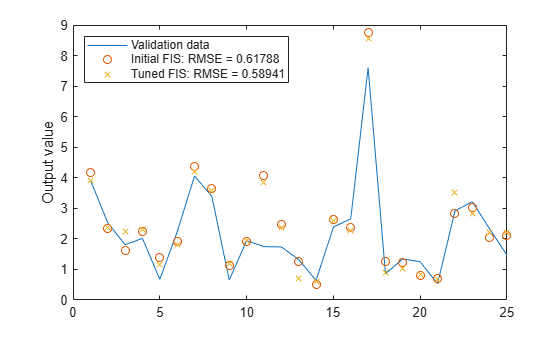This plot shows that the training error settles at about the 60th epoch point.

Plot the checking error, `chkErr`.

```figure plot(chkErr) title('Checking Error') xlabel('Number of Epochs') ylabel('Checking Error')```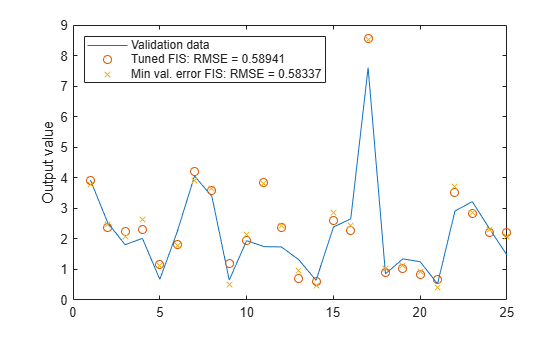The plot shows that the smallest value of the validation data error occurs at the 52nd epoch. After this point it increases slightly even as `anfis` continues to minimize the error against the training data all the way to the 200th epoch. Depending on the specified error tolerance, the plot also indicates the ability of the model to generalize the test data.

You can also compare the output of `fismat2` and `fistmat4` against the validation data, `chkdatout`.

```figure plot(chkdatout) hold on plot(chkfuzout4,'ob') plot(chkfuzout2,'+r')```